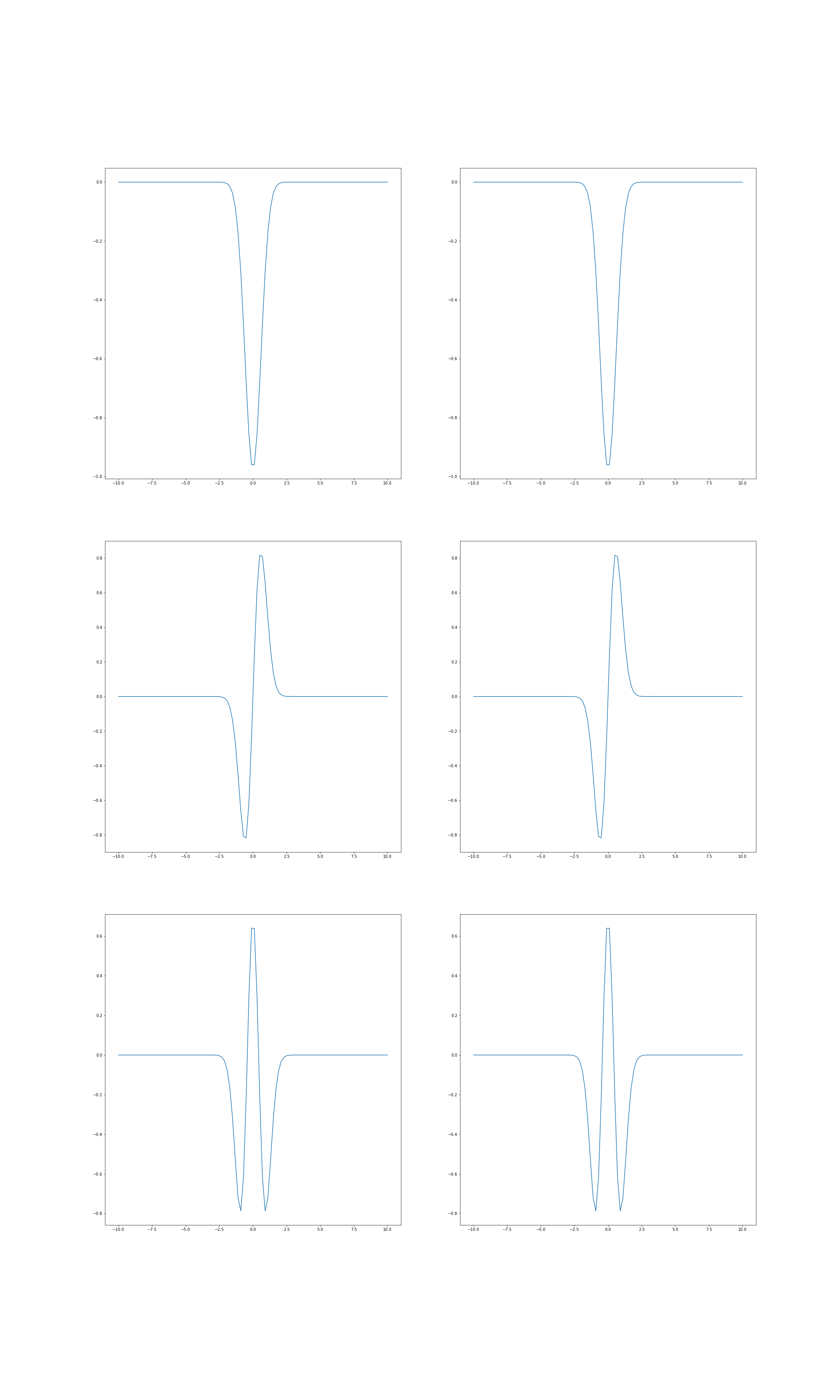Quantum Entanglement in Python

# Quantum Entanglement in Python

## Version

The releases of pyqentangle 2.x.x is incompatible with previous releases.

The releases of pyqentangle 3.x.x is incompatible with previous releases.

Since release 3.1.0, the support for Python 2.7 and 3.5 has been decomissioned. Since release 3.3.0, support for Python 3.6 is diminished, but that for Python 3.11 is added.

## Installation

This package can be installed using pip.

>>> pip install -U pyqentangle


To use it, enter

>>> import pyqentangle
>>> import numpy as np


## Schmidt Decomposition for Discrete Bipartite States

We first express the bipartite state in terms of a tensor. For example, if the state is |01>+|10>, then express it as

>>> tensor = np.array([[0., np.sqrt(0.5)], [np.sqrt(0.5), 0.]])


To perform the Schmidt decompostion, just enter:

>>> pyqentangle.schmidt_decomposition(tensor)
[(0.7071067811865476, array([ 0., -1.]), array([-1., -0.])),
(0.7071067811865476, array([-1.,  0.]), array([-0., -1.]))]


For each tuple in the returned list, the first element is the Schmidt coefficients, the second the component for first subsystem, and the third the component for the second subsystem.

## Schmidt Decomposition for Continuous Bipartite States

We can perform Schmidt decomposition on continuous systems too. For example, define the following normalized wavefunction:

>>> fcn = lambda x1, x2: np.exp(-0.5 * (x1 + x2) ** 2) * np.exp(-(x1 - x2) ** 2) * np.sqrt(np.sqrt(8.) / np.pi)


Then perform the Schmidt decomposition,

>>> modes = pyqentangle.continuous_schmidt_decomposition(biwavefcn, -10., 10., -10., 10., keep=10)


where it describes the ranges of x1 and x2 respectively, and keep=10 specifies only top 10 Schmidt modes are kept. Then we can read the Schmidt coefficients:

>>> list(map(lambda dec: dec, modes))
[0.9851714310094161,
0.1690286950361957,
0.02900073920775954,
0.004975740210361192,
0.0008537020544076649,
0.00014647211608480773,
2.51306421011773e-05,
4.311736522272035e-06,
7.39777032460608e-07,
1.2692567250688184e-07]


The second and the third elements in each tuple in the list decompositions are lambda functions for the modes of susbsystems A and B respectively. The Schmidt functions can be plotted:

>>> xarray = np.linspace(-10., 10., 100)

plt.subplot(3, 2, 1)
plt.plot(xarray, modes(xarray))
plt.subplot(3, 2, 2)
plt.plot(xarray, modes(xarray))

plt.subplot(3, 2, 3)
plt.plot(xarray, modes(xarray))
plt.subplot(3, 2, 4)
plt.plot(xarray, modes(xarray))

plt.subplot(3, 2, 5)
plt.plot(xarray, modes(xarray))
plt.subplot(3, 2, 6)
plt.plot(xarray, modes(xarray))## Reference

• Artur Ekert, Peter L. Knight, "Entangled quantum systems and the Schmidt decomposition", Am. J. Phys. 63, 415 (1995).

## Project details

This version3.3.13.3.03.2.33.2.23.2.13.2.03.1.113.1.103.1.93.1.83.1.73.1.63.1.53.1.43.1.33.1.23.1.13.1.03.0.13.0.02.0.01.1.01.0.31.0.21.0.11.0.00.210.200.190.180.170.160.150.140.130.120.110.1

Uploaded source